# KSEEB SSLC Class 10 Maths Solutions Chapter 15 Surface Areas and Volumes Ex 15.3

In this chapter, we provide KSEEB SSLC Class 10 Maths Solutions Solutions Chapter 15 Surface Areas and Volumes Ex 15.3 for English medium students, Which will very helpful for every student in their exams. Students can download the latest KSEEB SSLC Class 10 Maths Solutions Solutions Chapter 15 Surface Areas and Volumes Ex 15.3 pdf, free KSEEB SSLC Class 10 Maths Solutions Solutions Chapter 15 Surface Areas and Volumes Ex 15.3 pdf download. Now you will get step by step solution to each question.

### Karnataka State Syllabus Class 10 Maths SolutionsChapter 15 Surface Areas and Volumes Ex 15.3

(Take π =227 unless stated otherwise)

Question 1.
A metallic sphere of radius 4.2 cm is melted and recast into the shape of a cylinder of radius 6 cm. Find the height of the cylinder.
Solution:
Let the height of cylinder be ‘h’ cm.
Radius of cylinder, r = 6 cm.
i) Volume of metallic sphere, V = 43πr3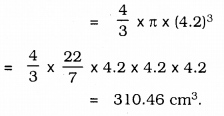ii) Volume of Cylinder, V = πr2h
227 × 36 × h = 310.46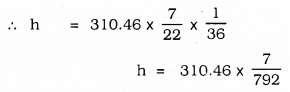∴ Height of cylinder is 2.74 cm.

Question 2.
Metallic spheres of radii 6 cm, 8 cm and 10 cm, respectively, are melted to form a single solid sphere. Find the radius of the resulting sphere.
Solution:
Let the radius of the resulting sphere be ‘r’ cm.
(i) Volume of 3 spheres having radii 8 cm and 10 cm.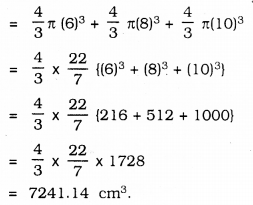(i) Volume of the resulting Sphere, V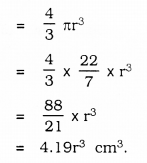∴ Volume of the resulting sphere = Volume of three spheres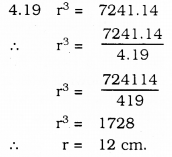∴ Radius of the resulting sphere is 12 cm.

Question 3.
A 20 m deep well with a diameter 7 m is dug and the earth from digging is evenly spread out to form a platform 22 m by 14 m. Find the height of the platform.
Solution:
Radius of well, ‘r’ = 3.5 m.
Height of well, h = 20 m.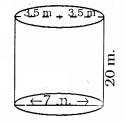(i) Volume of soil dug out of well, V = πr2h (∵ cylinder sphere)
= 227×(3.5)2×20
= =227×3510×3510×20
= 770m3
(ii) Length of platform, l = 22 m.
Height, h =?
Volume of Platform, V= l × b × h (∵ Cuboid)
= 22 × 14 × h
∴ Volume of Platform = Volume of soil dug out of well.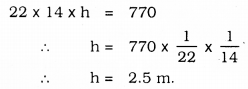∴ Height of platform is 2.5 m

Question 4.
A well of diameter 3 m is dug 14 m deep. The earth taken out of it has been spread evenly all around it in the shape of a circular ring of width 4 m to form an embankment. Find the height of the embankment.
Solution:
Let the height of the embankment be ‘h’ m.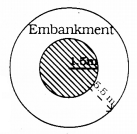Dimeter of well, d = 3 m.
Radius of well, r =1.5 m.
Height of well, h = 14 m.
Volume of an embankment = Volume of soil dug from well.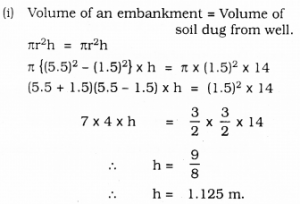∴ Height of embankment = 1.125 m.

Question 5.
A container shaped like a right cylinder diameter 12height 15 cm is full of ice cream. The ice cream is to be filled into cones of height 12 cm and diameter 6 cm, having a hemispherical shape on the top. Find the number of such cones cones which can be filled with ice cream.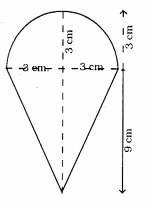Solution:
Diameter of right circular cylinder is 12 cm ➝ r = 6 cm.
Height: 15 cm. ➝ h = 15 cm.
Diameter of Cone, 6 cm. ➝ r = 3 cm.
Height 12 cm. ➝ h = 12 cm.
(i) Volume of the ice cream which has cone-shaped and hemisphere.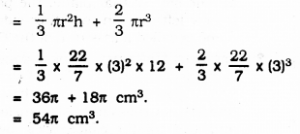(ii) Volume of cylindrical vessel, V = πr2h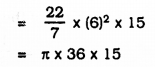Let number of cones be ‘n’,
n × 54π = π × 36 × 15
54n = 36 × 15
n=36×1554=54054
∴ n = 10
Ice cream can be filled in 10 cones.

Question 6.
How many silver coins, 1.75 cm. in diameter and of thickness 2 mm, must be melted to form a cuboid of dimensions 5.5 cm × 10 cm × 3.5 cm?
Solution:
Diameter of the silver coins is 1.75 cm.
Thickness = 2 mm.
Measurement of cuboid: 5.5 × 10 × 3.5 cm.
∴ Let the required silver coins be ‘n’,
Volume of ‘n’ coins = Volume of cuboid
πr2h = l × b × h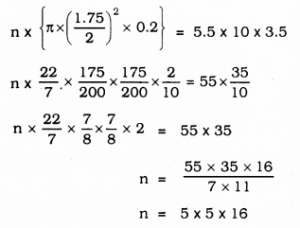∴ n = 400
∴ 400 silver coins are required.

Question 7.
A cylindrical bucket, 32 cm high and with a radius of base 18 cm, is filled with sand. This bucket is emptied on the ground and a conical heap of sand is formed. If the height of the conical heap is 24 cm, find the radius and slant height of the heap.
Solution:
Height of cylindrical bucket, h = 32 cm.
Height of conical heap of sand = 24 cm.
Slant height, l =?
Volume of the conical heap of sand = Volume of Cylinder.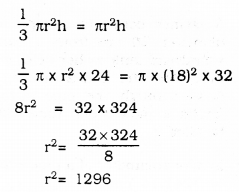Radius of Cone, r = 36 cm.
Slant height of cone, l = h2+r2−−−−−−√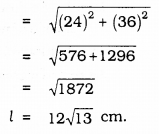∴ Radius of cone, r = 36 cm.
Slant height of cone, h = 1213−−√ cm

Question 8.
Water in a canal, 6 m wide and 1.5 m deep, is flowing with a speed of 10 km/h. How much area will it irrigate in 30 minutes, if 8 cm of standing water is needed?
Solution:
Breadth of a canal, b = 6 m.
Height, h = 1.5 m
Speed of water =10 km/hr.
Quantity of water = 8 cm.
Quantity of water flowing in 30 minutes?
Volume of water flowing in 60 minutes,
= (10 × 1000) × 6 × 1.5 cm3.
( ∵ Speed = 10 km/hr.; 10 × 1000 m/hr.)
Volume of water flowing in 30 minutes,
= 10000×9×3060m3
∴ Let the area of land be ‘x’ sq.m.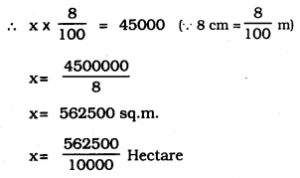x= 562500 sq.m.

Question 9.
A farmer connects a pipe of internal diameter 20 cm from a canal into a cylindrical tank in her field, which is 10 m in diameter and 2 m deep. If water flows through the pipe at the rate of 3 km/h, in how much time will the tank be filled?
Solution:
Internal diameter of a pipe = 20 cm.
Radius of pipe =10 cm. = 110m
Diameter of cylindrical tank = 10 m; r=5 m.
Depth of cylindrical tank = 2 m.
Speed of water is 3 km/hr.
Time required to fill the water tank =?
Speed of water = 3 km/hr.
= 300060 m/minute
= 50 m/min.
Let the time required to fill the tank be n’ minutes.
∴ Flowing water in ‘n’ minutes = Volume of the water tank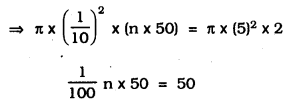∴ n = 100 minutes
∴ Time required to fill the water tank = 100 minutes

All Chapter KSEEB Solutions For Class 10 Maths

—————————————————————————–

All Subject KSEEB Solutions For Class 10

*************************************************

I think you got complete solutions for this chapter. If You have any queries regarding this chapter, please comment on the below section our subject teacher will answer you. We tried our best to give complete solutions so you got good marks in your exam.

If these solutions have helped you, you can also share kseebsolutionsfor.com to your friends.

Best of Luck!!